# 15. Polynomial Class

## IntroductionIf you have been to highschool, you will have encountered the terms polynomial and polynomial function. This chapter of our Python tutorial is completely on polynomials, i.e. we will define a class to define polynomials. The following is an example of a polynomial with the degree 4:

$$p(x) = x^4 - 4 \cdot x^2 + 3 \cdot x$$

We will see that you can calculate with polynomials in the same way as with integers and floats. Operations which can also be implemented in Python. We will define various arithmetic operations for polynomials in our class, like addition, subtraction, multiplication and division. Our polynomial class will also provide means to calculate the derivation and the integral of polynomials. We will not miss out on plotting polynomials.

There is a lot of beaty in polynomials and above all in how they can be implemented as a Python class. We like to say thanks to Drew Shanon who granted us permission to use his great picture, treating math as art!

Live Python training

Enjoying this page? We offer live Python training courses covering the content of this site.

Enrol here

## Short Mathematical Introduction

We will only deal with polynomial in a single indeterminate (also called variable) x. A general form of a polynomial in a single indeterminate looks like this:

$$a_n \cdot x^n + a_{n-1} \cdot x^{n-1} + \ldots + a_2 \cdot x^2 + a_1 \cdot x + a_0$$

where $a_0, a_1, ... a_n$ are the constants - non-negative integers - and $x$ is the indeterminate or variable. The term "indeterminate" means that $x$ represents no particular value, but any value may be substituted for it.

This expression is usually written with the summation operator:

$$\sum_{k=0}^{n} a_k \cdot x^k = a_n \cdot x^n + a_{n-1} \cdot x^{n-1} + \ldots + a_2 \cdot x^2 + a_1 \cdot x + a_0$$

A polynomial function is a function that can be defined by evaluating a polynomial. A function f of one argument can be defined as:

$$f(x) = \sum_{k=0}^{n} a_k \cdot x^k$$

## Polynomial Functions with Python

It's easy to implement polynomial functions in Python. As an example we define the polynomial function given in the introduction of this chapter, i.e. $p(x) = x^4 - 4 \cdot x^2 + 3 \cdot x$

The Python code for this polynomial function looks like this:

def p(x):
return x**4 - 4*x**2 + 3*x


We can call this function like any other function:

for x in [-1, 0, 2, 3.4]:
print(x, p(x))


### OUTPUT:

-1 -6
0 0
2 6
3.4 97.59359999999998


We can plot the function in a simple way with Matplotlib:

import numpy as np
import matplotlib.pyplot as plt

X = np.linspace(-3, 3, 50, endpoint=True)
F = p(X)
plt.plot(X,F)

plt.show()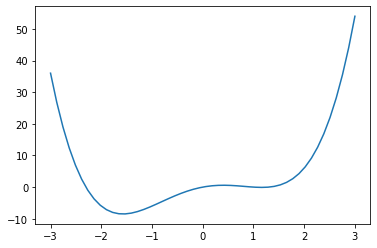Live Python training

Enjoying this page? We offer live Python training courses covering the content of this site.

Upcoming online Courses

Enrol here

## Polynomial Class in Python

We will define now a class for polynomial functions in Python. We will build on an idea which we have developed in the chapter on decorators of our Python tutorial. We introduced polynomial factories.

A polynomial is uniquely determined by its coefficients. This means, an instance of our polynomial class needs a list or tuple to define the coefficients.

class Polynomial:

def __init__(self, *coefficients):
""" input: coefficients are in the form a_n, ...a_1, a_0
"""
self.coefficients = list(coefficients) # tuple is turned into a list

def __repr__(self):
"""
method to return the canonical string representation
of a polynomial.
"""
return "Polynomial" + str(tuple(self.coefficients))



We can the instantiate the polynomial of our previous example polynomial function like this:

p = Polynomial(1, 0, -4, 3, 0)
print(p)

p2 = eval(repr(p))
print(p2)


### OUTPUT:

Polynomial(1, 0, -4, 3, 0)
Polynomial(1, 0, -4, 3, 0)


Having defined the canonical string representation of a Polynomial with the repr function, we also want to define an output which could be used to create a version which is more friendly for people. We write a LaTex represention which can be used to print the function in a beatiful way:

class Polynomial:

def __init__(self, *coefficients):
""" input: coefficients are in the form a_n, ...a_1, a_0
"""
self.coefficients = list(coefficients) # tuple is turned into a list

def __repr__(self):
"""
method to return the canonical string representation
of a polynomial.
"""
return "Polynomial" + str(tuple(self.coefficients))

def __str__(self):

def x_expr(degree):
if degree == 0:
res = ""
elif degree == 1:
res = "x"
else:
res = "x^"+str(degree)
return res

degree = len(self.coefficients) - 1
res = ""

for i in range(0, degree+1):
coeff = self.coefficients[i]
# nothing has to be done if coeff is 0:
if abs(coeff) == 1 and i < degree:
# 1 in front of x shouldn't occur, e.g. x instead of 1x
# but we need the plus or minus sign:
res += f"{'+' if coeff>0 else '-'}{x_expr(degree-i)}"
elif coeff != 0:
res += f"{coeff:+g}{x_expr(degree-i)}"

return res.lstrip('+')    # removing leading '+'

polys = [Polynomial(1, 0, -4, 3, 0),
Polynomial(2, 0),
Polynomial(4, 1, -1),
Polynomial(3, 0, -5, 2, 7),
Polynomial(-42)]

# output suitable for usage in LaTeX:
for count, poly in enumerate(polys):
print(f"$p_{count} = {str(poly)}$")


### OUTPUT:

$p_0 = x^4-4x^2+3x$
$p_1 = 2x$
$p_2 = 4x^2+x-1$
$p_3 = 3x^4-5x^2+2x+7$
$p_4 = -42$


If we use this in LaTeX it will look like this:

$p_0 = x^4-4x^2+3x$

$p_1 = 2x$

$p_2 = 4x^2+x-1$

$p_3 = 3x^4-5x^2+2x+7$

$p_4 = -42$

So far we have defined polynomials, but what we actually need are polynomial functions. For this purpose, we turn instances of the Polynomial class into callabes, which are callable class instances. To do this, we define method call:

class Polynomial:

def __init__(self, *coefficients):
""" input: coefficients are in the form a_n, ...a_1, a_0
"""
self.coefficients = list(coefficients) # tuple is turned into a list

# The __repr__ and __str__ method can be included here,
# but is not necessary for the immediately following code

def __call__(self, x):
res = 0
for index, coeff in enumerate(self.coefficients[::-1]):
res += coeff * x** index
return res


It is now possible to call an instance of our class like a function. We call it with an argument and the instance, - which is a callable, - behaves like a polynomial function:

p = Polynomial(3, 0, -5, 2, 1)
print(p)

for x in range(-3, 3):
print(x, p(x))


### OUTPUT:

<__main__.Polynomial object at 0x7fa46c2fa8e0>
-3 193
-2 25
-1 -3
0 1
1 1
2 33


Just for fun, let us plot the previously defined function:

import matplotlib.pyplot as plt

X = np.linspace(-1.5, 1.5, 50, endpoint=True)
F = p(X)
plt.plot(X, F)

plt.show()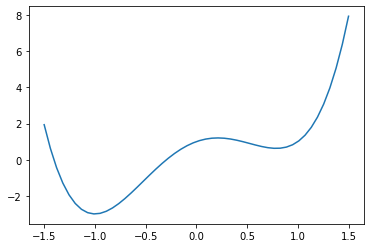Before we further refine our class, let us use a numerically more efficient variant for the calculation of polynomials. We will use this algorithm in our __call__ method. We introduced this variant in our chapter on decorators.

Every polynomial

$f(x) = \sum_{k=0}^{n} a_k \cdot x^k$

can be also written in the form

$f(x) = (a_n x + x_{n-1})x + \cdot + a_1)x + a_0$

To emperically see that they are equivalent, we rewrite our class definition, but call it Polynomial2 so that we can use both versions:

class Polynomial2:

def __init__(self, *coefficients):
""" input: coefficients are in the form a_n, ...a_1, a_0
"""
self.coefficients = list(coefficients) # tuple is turned into a list

# The __repr__ and __str__ method can be included here,
# but is not necessary for the immediately following code

def __call__(self, x):
res = 0
for coeff in self.coefficients:
res = res * x + coeff
return res


We will test both classes by comparing the results:

p1 = Polynomial(-4, 3, 0)
p2 = Polynomial2(-4, 3, 0)
res = all((p1(x)==p2(x) for x in range(-10, 10)))
print(res)


### OUTPUT:

True


It is possible to define addition and subtractions for polynomials. All we have to do is to add or subtract the coefficients with the same exponents from both polynomials.

If we have polynomial functions $f(x) = \sum_{k=0}^{n} a_k \cdot x^k$ and $g(x) = \sum_{k=0}^{m} b_k \cdot x^k$, the addition is defined as

$$(f+g)(x) = \sum_{k=0}^{\max(n, m)} (a_k + b_k) \cdot x^k$$

and correspondingly subtraction is defined as

$$(f-g)(x) = \sum_{k=0}^{\max(n, m)} (a_k - b_k) \cdot x^k$$

Before we can add the methods __add__ and __sub__, which are necessary for addition and subtraction, we add a generator zip_longest. It works similar to zip for two parameters, but it does not stop if one of the iterators is exhausted but uses "fillvalue" instead.

def zip_longest(iter1, iter2, fillvalue=None):

for i in range(max(len(iter1), len(iter2))):
if i >= len(iter1):
yield (fillvalue, iter2[i])
elif i >= len(iter2):
yield (iter1[i], fillvalue)
else:
yield (iter1[i], iter2[i])
i += 1

p1 = (2,)
p2 = (-1, 4, 5)
for x in zip_longest(p1, p2, fillvalue=0):
print(x)


### OUTPUT:

(2, -1)
(0, 4)
(0, 5)


We will add this generator to our class as a static method. We are now capable of adding the __add__ and __sub__ methods as well. We will need the generator zip_longest from itertools. zip_longest takes and arbitrary number of iterables and a keyword parameter fillvalue. It creates an iterator that aggregates elements from each of the iterables. If the iterables don't have the same length, missing values are filled-in with fillvalue. Iteration continues until the longest iterable is exhausted.

import numpy as np
import matplotlib.pyplot as plt
from itertools import zip_longest

class Polynomial:

def __init__(self, *coefficients):
""" input: coefficients are in the form a_n, ...a_1, a_0
"""
self.coefficients = list(coefficients) # tuple is turned into a list

def __repr__(self):
"""
method to return the canonical string representation
of a polynomial.

"""
return "Polynomial" + str(self.coefficients)

def __call__(self, x):
res = 0
for coeff in self.coefficients:
res = res * x + coeff
return res

def degree(self):
return len(self.coefficients)

c1 = self.coefficients[::-1]
c2 = other.coefficients[::-1]
res = [sum(t) for t in zip_longest(c1, c2, fillvalue=0)]
return Polynomial(*res[::-1])

def __sub__(self, other):
c1 = self.coefficients[::-1]
c2 = other.coefficients[::-1]

res = [t1-t2 for t1, t2 in zip_longest(c1, c2, fillvalue=0)]
return Polynomial(*res[::-1])

p1 = Polynomial(4, 0, -4, 3, 0)
p2 = Polynomial(-0.8, 2.3, 0.5, 1, 0.2)

p_sum = p1 + p2
p_diff = p1 - p2

X = np.linspace(-3, 3, 50, endpoint=True)
F1 = p1(X)
F2 = p2(X)
F_sum = p_sum(X)
F_diff = p_diff(X)
plt.plot(X, F1, label="F1")
plt.plot(X, F2, label="F2")
plt.plot(X, F_sum, label="F_sum")
plt.plot(X, F_diff, label="F_diff")

plt.legend()
plt.show()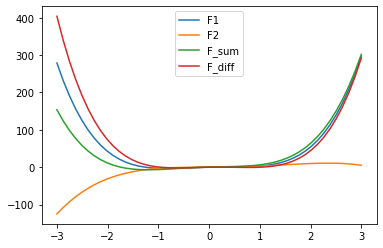It is incredibly easy to add differentiation to our class. Mathematically, it is defined as

$$f'(x) = \sum_{k=1}^{n} k \cdot a_k \cdot x^{k-1}$$

if

$$f(x) = \sum_{k=0}^{n} a_k \cdot x^k$$

This can be easily implemented in our method 'derivative':

import numpy as np
import matplotlib.pyplot as plt
from itertools import zip_longest

class Polynomial:

def __init__(self, *coefficients):
""" input: coefficients are in the form a_n, ...a_1, a_0
"""
self.coefficients = list(coefficients) # tuple is turned into a list

def __repr__(self):
"""
method to return the canonical string representation
of a polynomial.

"""
return "Polynomial" + str(self.coefficients)

def __call__(self, x):
res = 0
for coeff in self.coefficients:
res = res * x + coeff
return res

def degree(self):
return len(self.coefficients)

c1 = self.coefficients[::-1]
c2 = other.coefficients[::-1]
res = [sum(t) for t in zip_longest(c1, c2, fillvalue=0)]
return Polynomial(*res)

def __sub__(self, other):
c1 = self.coefficients[::-1]
c2 = other.coefficients[::-1]

res = [t1-t2 for t1, t2 in zip_longest(c1, c2, fillvalue=0)]
return Polynomial(*res)

def derivative(self):
derived_coeffs = []
exponent = len(self.coefficients) - 1
for i in range(len(self.coefficients)-1):
derived_coeffs.append(self.coefficients[i] * exponent)
exponent -= 1
return Polynomial(*derived_coeffs)

def __str__(self):

def x_expr(degree):
if degree == 0:
res = ""
elif degree == 1:
res = "x"
else:
res = "x^"+str(degree)
return res

degree = len(self.coefficients) - 1
res = ""

for i in range(0, degree+1):
coeff = self.coefficients[i]
# nothing has to be done if coeff is 0:
if abs(coeff) == 1 and i < degree:
# 1 in front of x shouldn't occur, e.g. x instead of 1x
# but we need the plus or minus sign:
res += f"{'+' if coeff>0 else '-'}{x_expr(degree-i)}"
elif coeff != 0:
res += f"{coeff:+g}{x_expr(degree-i)}"

return res.lstrip('+')    # removing leading '+'

p = Polynomial(-0.8, 2.3, 0.5, 1, 0.2)

p_der = p.derivative()

X = np.linspace(-2, 3, 50, endpoint=True)

F = p(X)
F_derivative = p_der(X)
plt.plot(X, F, label="F")
plt.plot(X, F_derivative, label="F_der")

plt.legend()
plt.show()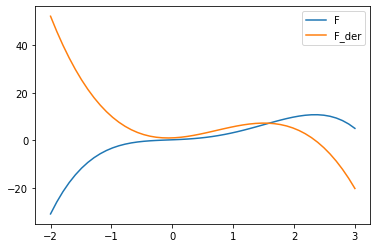Let's use in the following Python example addition and subtraction now:

p = Polynomial(1, 2, -3, 4, -5)

p2 = Polynomial(3, 2, -3, 4, 3)

(p + p2)


### OUTPUT:

Polynomial[-2, 8, -6, 4, 4]

import matplotlib.pyplot as plt
plt.plot(X, p(X), label="p")
plt.plot(X, p2(X), label="p2")
plt.plot(X, (p + p2)(X), label="p + p2")
plt.plot(X, (p - p2)(X), label="p - p2")

plt.legend()
plt.show()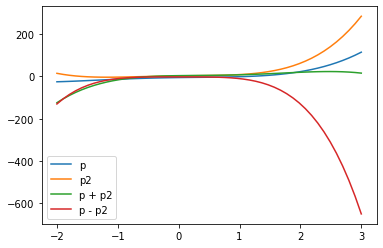Live Python training

Enjoying this page? We offer live Python training courses covering the content of this site.

Upcoming online Courses

Enrol here